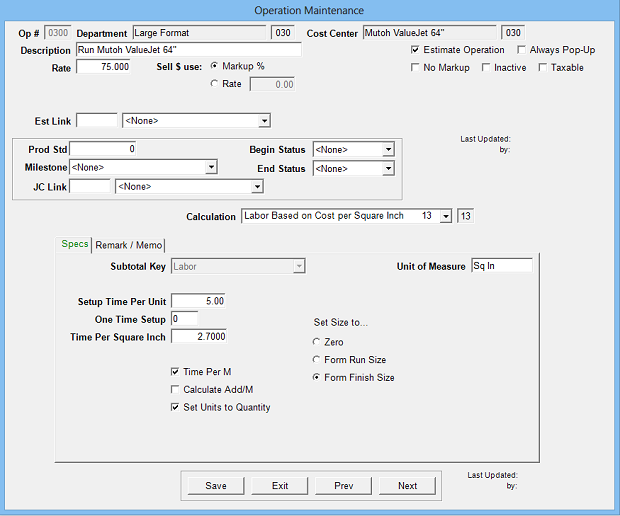Estimates the cost and time of labor by the square inch.  Commonly used for Large Format estimates.  A translation from square feet per hour (or minute) to minutes per square inch may be required to setup.

Operation Setup Requirements:

1. Description
2. Rate: Cost per hour
3. Sell \$ use: Markup % (recommended) or alternate sell rate
4. Calc: 13
5. Sub Key: Labor
6. Setup Time per Unit: Minutes for each unit
7. One Time Setup: Base setup minutes
8. Time per Square inch: Minutes for each square inch
9. Time per M: Option to make time per 1000 units
11. Set Units to Quantity Option
12. Set size to: Zero (editable), Form Run Size or Form Finish SizeEstimate Specification Input Prompts:

1. Length x Width (inches).  Not editable if set to form run size or finish size

2. Units

3. J%

4. Remarks

5. MemoCalculation:

Length x Width = Square Inches per Unit

Units x Square Inches per Unit = Total Square Inches

(Base Setup Min) + (Units x Min per Unit) + (Total Sq Inches x Min per Square Inch) = Total Min

If Time per M option selected, divide Total Min by 1000

(Total Minutes / 60) x J% = Estimated Hours

Estimated Hours x Rate = Cost \$

Cost \$ x Markup % = Markup \$

Cost \$ + Markup \$ = Sell \$